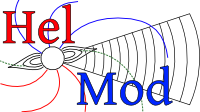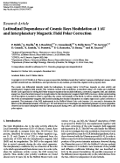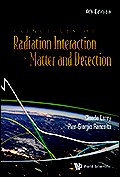HelMod-4 The Heliospheric Modulation Model Online Calculator Version TestThe Interplaneraty Magnetic Field (IMF) is commonly described using the so-called Parker Spiral [see e.g. Parker, 1958; Bobik et al., 2012; Chapter 8 in Leroy & Rancoita, 2016 and reference there in]. This description is valid for i) a constant solar rotation with angular velocity ($$\omega$$), ii) a simple spherically symmetric emission of the SW
and iii) a constant (or approaching an almost constant) SW speed ($$V_{\textrm{sw}}$$) at larger radial distances ($$r$$), e.g., for $$r > r_b \approx 10$$R$$_\odot$$ (where R$$_\odot$$ is the Solar radius), since beyond $$r_b$$
the wind speed varies slowly with the distance.

In 1989, Jokipii and Kota have argued that the solar surface is not a smooth surface, but a granular turbulent surface that keeps changing with time, especially in the polar regions. This turbulence may cause temporal deviations from the smooth Parker geometry creating transverse components in the field. Thus, the magnitude of the mean magnetic field at the poles is greater than in the case of the smooth magnetic field of a pure Parker spiral. This was accounted by the introduction of a perturbation parameter [$$\delta(\theta)$$] which amplifies the field strength at large radial distances.

In the present treatment, the heliosphere is divided into polar regions and a equatorial region where different description of IMF are applied. In the equatorial region the Parker's IMF, is used:

\begin{equation}\vec B_{Par} = \dfrac{A}{r^{2}}\left[\vec e_{r}-\dfrac{\omega(r-r_b)\sin\theta}{V_{sw}}\vec e_{\varphi}\right]\left[1-2H\left(\theta-\theta'\right)\right] \end{equation}

while in the polar regions we used a modified IMF as described in Bobik et al. 2013:
\begin{equation}\label{IMFparpol}
\vec B_{Pol} = \dfrac{A}{r^{2}}\left[\vec e_{r}+\dfrac{r}{r_b}\delta(\theta)\vec e_{\theta}-\dfrac{\omega(r-r_b)\sin\theta}{V_{sw}}\vec e_{\varphi}\right]\left[1-2H\left(\theta-\theta'\right)\right]
\end{equation}

where $$A$$ is a coefficient that determines the IMF polarity and allows $$|\vec B_{Par} |$$ to be equal to $$B_\oplus$$, i.e., the value of the IMF at Earth's orbit as extracted from NASA/GSFC's OMNI data set;  $$\vec e_r$$ and $$\vec e_\varphi$$ are unit vector components in the radial and azimuthal directions, respectively; $$\theta$$ is the colatitude (polar angle); $$\theta'$$ is the polar angle determining the position of the Heliospheric Current Sheet (HCS); $$H$$ is the Heaviside function: thus, $$[1-2H(\theta-\theta')]$$ allows $$\vec B_{Par}$$ to change sign in the two regions above and below the HCS of the heliosphere; finally,
\begin{equation}
\Gamma=\tan\Psi=\frac{\omega (r-r_b) \sin\theta}{V_{\textrm{sw}}}
\end{equation}
with $$\Psi$$  the spiral angle and $$\Omega$$ is assumed to be independent of the heliographic latitude and equal to the sidereal rotation at the Sun's equator.

In order to have a divergence-free magnetic-field Bobik et al. 2013 require that the perturbation factor [$$\delta(\theta)$$] has to be:
\begin{equation}\label{deltatheta}
\delta(\theta)=\frac{\delta_{m}}{\left[1-2H\left(\theta-\theta'\right)\right]\sin\theta},
\end{equation}
where $$\delta_{m}$$ is the minimum perturbation factor of the field. The perturbation parameter is let to grow with decreasing of the colatitude.

#### BibliographyBobik, P., Boella, G., Boschini, M.~J., Consolandi, C., Della Torre, S., Gervasi, M., Grandi, D., Kudela, K., Pensotti, S., Rancoita P.G. and Tacconi, M. (2012) Astrophys. J., 745:132 .Bobik, P., Boella, G., Boschini, M.~J., Consolandi, C., Della Torre, S., Gervasi, M., Grandi, D., Kudela, K., Pensotti, S., Rancoita P.G., Rozza, D. and Tacconi, M. (2013).  Advances in Astronomy, vol. 2013, id.793072C. Leroy and P.G. Rancoita (2016), Principles of Radiation Interaction in Matter and Detection - 4th Edition -, World Scientific. Singapore, ISBN-978-981-4603-18-8 (printed) Jokipii, J. R. and Kota, J. (1989), Geophysical Research Letters, vol. 16, p. 1-4. Parker, E. N. (1958), Astrophysical Journal, vol. 128, p.664